# Integral operator

(diff) ← Older revision | Latest revision (diff) | Newer revision → (diff)

A mappingin which the law of the correspondenceis given by an integral. An integral operator is sometimes called an integral transformation. Thus, for Urysohn's integral operator (cf. Urysohn equation)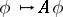, the law of the correspondenceis given by the integral (or the operatoris generated by the integral)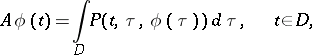(1)

whereis a given measurable set of finite Lebesgue measure in a finite-dimensional space and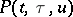,,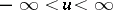, is a given measurable function. It is assumed thatandare functions satisfying conditions that ensure the existence of the integral in (1) in the sense of Lebesgue. Ifis a non-linear function in, then (1) is an example of a non-linear integral operator. If, on the other hand,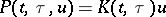, then (1) takes the form(2)

The operator generated by the integral in (2), or simply the operator (2), is called a linear integral operator, and the functionis called its kernel (cf. also Kernel of an integral operator).

The kernelis called a Fredholm kernel if the operator (2) corresponding tois completely continuous (compact) from a given function spaceinto another function space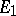. In this case, the operator (2) is called a Fredholm integral operator frominto.

Linear integral operators are often considered in the following function spaces:, the space of continuous functions on a bounded closed set, and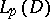, the space of functions onwhose-th powers are integrable. In the first case (2) is called a Fredholm operator in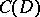(i.e. frominto) ifis continuous on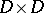(is then called a continuous kernel). The operator (2) is a Fredholm operator in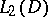(frominto) ifis measurable onand if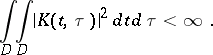(3)

Such a kernel is called an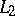-kernel.

The adjoint of (2), in the complex function space, with kernel satisfying (3), is the integral operator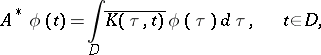(4)

where the bar means transition to the complex conjugate. Ifis a Hermitian (symmetric) kernel (i.e.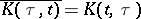), then the corresponding Fredholm operator (2) coincides with its adjoint (4). Operators with this property are called self-adjoint (cf. Self-adjoint operator). A Fredholm operator with a symmetric kernel is called a Hilbert–Schmidt integral operator.

If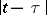denotes the distance between two pointsandin the-dimensional Euclidean space and if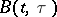is a bounded measurable function on, then a kernel of the form(5)

is called a kernel of potential type, and an operator (2) with such a kernel is called an integral operator of potential type. The kernel (5) is also called a polar kernel, or a kernel with weak singularity, while the corresponding operator (2) is called an integral operator with weak singularity.

Ifis a continuous function on, the corresponding integral operator with weak singularity is completely continuous in, while ifis a bounded measurable function on, the corresponding operator is completely continuous in.

If the kerneland the-dimensional setare such that the integral (2) does not exist in the sense of Lebesgue, but does exist in the sense of the Cauchy principal value, then the integral (2) is called an-dimensional singular integral (cf. also Singular integral). The operator generated by it is called an-dimensional singular integral operator, or a one-dimensionalor multi-dimensional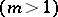singular integral operator.

If a curvelies in the complex-plane, then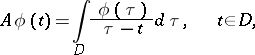(6)

where the integral is understood in the sense of the Cauchy principal value, generates a continuous integral operatorin the space of functions satisfying a Hölder condition (ifis a simple closed curve) or in,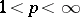(ifis a Lyapunov curve, cf. also Lyapunov surfaces and curves). The operator (6) is called a singular Cauchy operator.

Suppose that two Lebesgue-measurable functionsandare given on the real axis. If for almost-all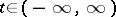the integral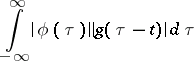exists, then one can define the function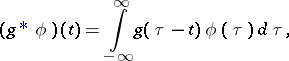(7)

called the convolution ofand. Ifis fixed, (7) defines an operator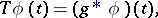(8)

which is called the convolution integral operator (or convolution integral transform, cf. also Convolution transform) with kernel.

If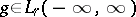,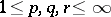, and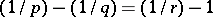, then (8) is a continuous operator frominto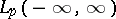. Under corresponding conditions, convolution integral operations are used on a semi-axis as well as on a finite interval.

Apart from the integral operators above, concrete classes of integral operators have been studied, e.g. the integral transforms of Fourier, Laplace, Bessel, Mellin, Hilbert, etc.

How to Cite This Entry:
Integral operator. Encyclopedia of Mathematics. URL: http://encyclopediaofmath.org/index.php?title=Integral_operator&oldid=15387
This article was adapted from an original article by B.V. Khvedelidze (originator), which appeared in Encyclopedia of Mathematics - ISBN 1402006098. See original article